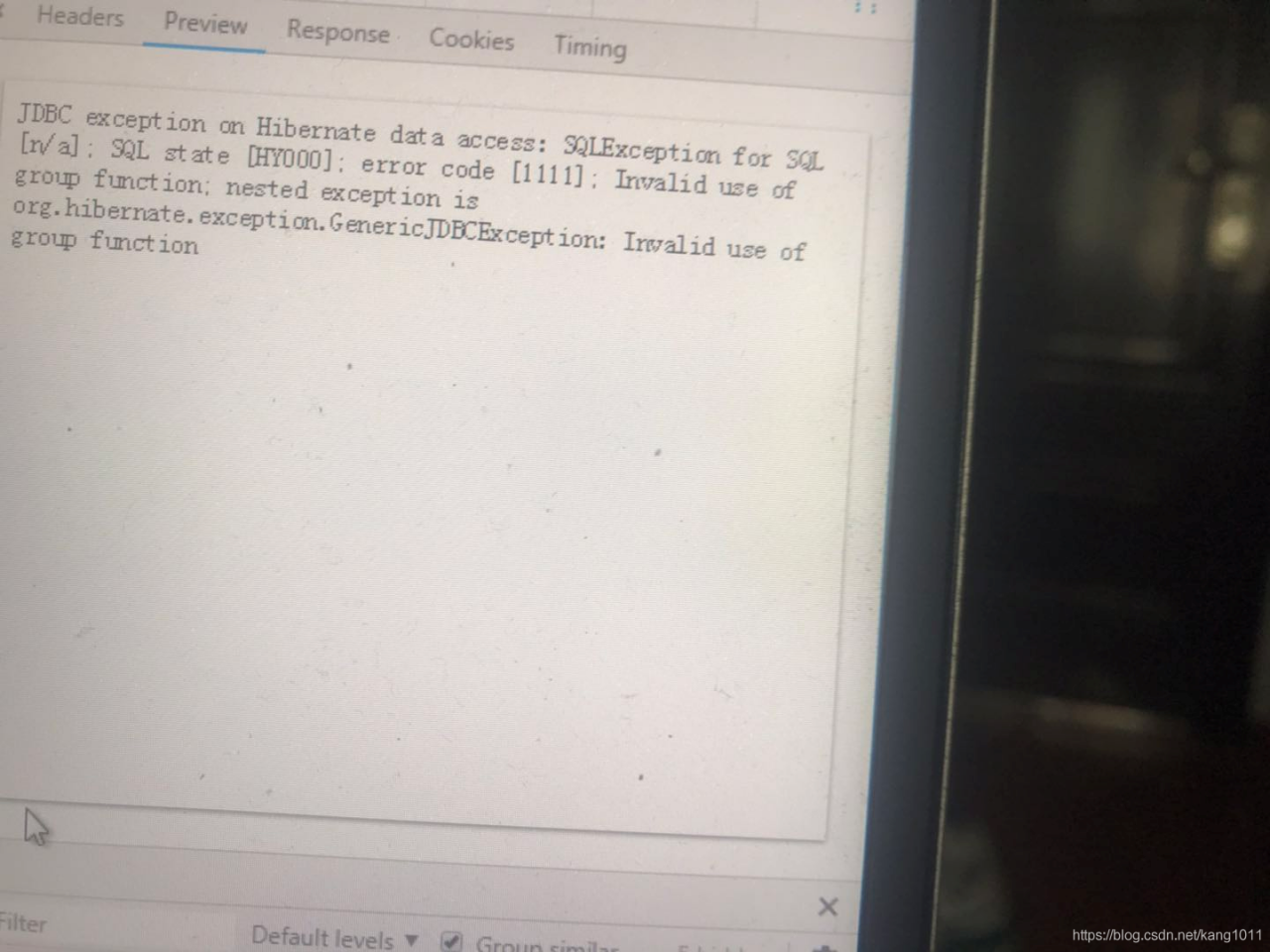# Mysql :error 1111. Invalid use of group function

Invalid use of group function Invalid use of group function
The phenomenon of

Oracle executes double-layer sum without Invalid use of group function, executes the same SQL to mysql, and Invalid use of group functionfind the cause
check correct

``````SELECT [DISTINCT|DISINCTROW|ALL] select_expression,... -- Query results
[FROM table_references -- specify the table for the query
[WHERE where_definition] -- where clause, the filtering condition of the query data
[GROUP BY col_name,...] -- group the query results [that match the where clause]
[HAVING where_definition] -- condition on the grouped results
[ORDER BY{unsigned_integer | col_name | formula} [ASC | DESC],...] -- Sort the query results
[LIMIT [offset,] rows] -- Limit the number of rows to be displayed for a query
[PROCEDURE procedure_name] -- query the result set data returned by the procedure
]
``````

Take a closer look at SQL to locate the two-layer SUM cause

Modify separate SUM, problem solved, the outermost package of a layer